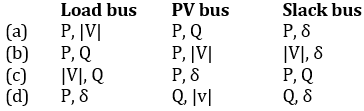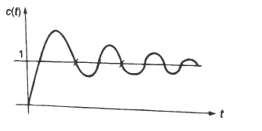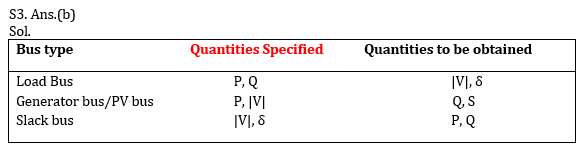Engineering Jobs   »   Electrical Engineering quizs   »   GATE 2022. GATE ELECTRICAL, GATE ELECTRICAL...

# GATE’22 EE: Daily Practices Quiz 13-Sep-2021

GATE 2022 Important Dates: Organizing Institute for GATE 2022 is the Indian Institute of Technology Kharagpur. For GATE 2022 Exam IIT Kharagpur has released the official notification and Important dates regarding the GATE 2022 Exam. Aspirants preparing for GATE 2022 must check the details regarding GATE 2022 Important Dates. Read the complete article below for the information related to GATE 2022 Important dates.

GATE’22 EE: Daily Practices Quiz 13-Sep-2021

Each question carries 2 marks
Negative marking: 2/3 mark

Total Questions: 05

Total marks: 10
Time: 12 min.

Q1. A sensor requires 30 s to indicate 90% of the response to a step input. If the sensor is a first – order system, the time constant is
[given,log_e (0.1)= -2.3]
(a) 15 s
(b) 13 s
(c) 21 s
(d) 28 s

Q2. Consider the time response of a second – order system with damping coefficient less than 1 to a unit step input:
1. It is over damped.
2. It is periodic function.
3. Time duration between any two consecutive values of 1 is the same.
Which of the above statements is/are correct?
(a) 1, 2 and 3
(b) 1 only
(c) 2 only
(d) 3 only

Q3. Buses for load flow studies are classified as:
2. PV bus
3. Slack bus
Which one of the following is the correct combination of the pair of quantities specified having their usual meaning for different buses?Q4. If the applied voltage of a certain transformer is increased by 50 % and the frequency is reduced to 50 % (assuming that the magnetic circuit remains unsaturated), the maximum core flux will
(a) Remains the same as original
(b) Change to three times the original value
(c) Change to 0.5 times the original value
(d) Change to 1.5 times the original value

Q5. What happened to the speed when the flux is reduced by 10 % in a 200 V DC shunt motor having an armature resistance of 0.2 Ω carrying a current of 50 A and running at 960 rpm prior to weakening of field. The total torque may be assumed constant & neglect losses….
(a) 1250 rpm
(b) 576 rpm
(c) 920 rpm
(d) 1066 rpm

SOLUTIONS
S1. Ans.(b)
Sol. Step response of first order system,
c(t)=1-e^(-t\/τ)
0.9=1-e^(-30\/τ)
⇒ e^(-30\/τ)=0.1;
⇒ τ= -30/(log_e (0.1) )=30/2.3 ▭(τ=13 sec)

S2. Ans.(d)
Sol.For an underdamped system ξ < 1; oscillation is damped by frequency.
ωd=ωn √(1-ξ^2 )S4. Ans.(b)
Sol. ϕ α V/f
ACQ: the applied voltage of a certain transformer is increased by 50 % and the frequency is reduced to 50 %.
∴ϕ^’=(1.5 V_1)/(0.5 f_1 )=3ϕ.

S5. Ans.(d)
Sol. Torque (T)=kϕN=constant
ACQ: T_=T2
∴ϕ1 N1=ϕ2 N2
∴N2=(ϕ1 N1)/ϕ2 =(ϕ1×960)/(0.9ϕ1 ) (Flux is reduced by 10%)
⇒N2=1066 rpm.

Sharing is caring!

Thank You, Your details have been submitted we will get back to you.